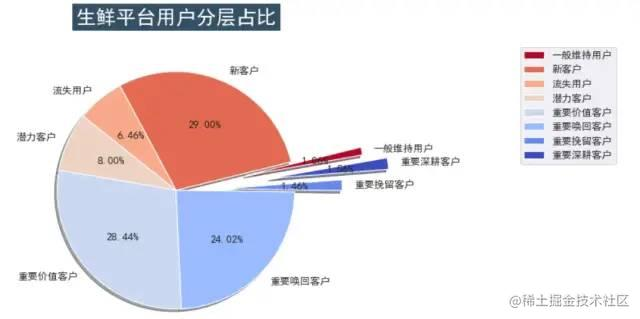# 最详细的 Python 结合 RFM 模型实现用户分层实操案例！｜Python 主题月

### 前言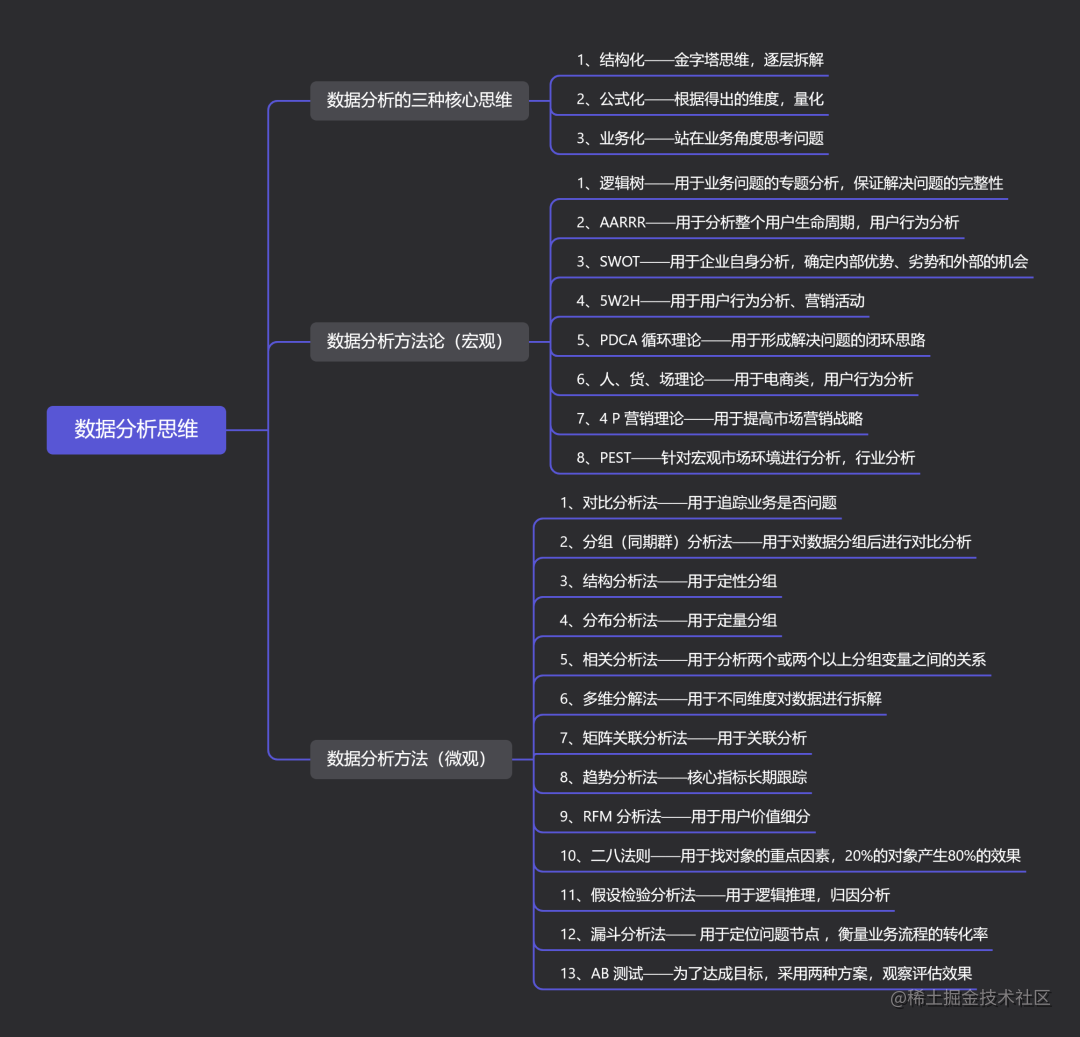### 一、整体分析流程

• 分析目的：用户分类
• 数据获取：Excel 数据
• 清洗加工：Excel、Python
• 建立模型：RFM
• 数据可视化
• 结论与建议

### 二、RFM 模型的理解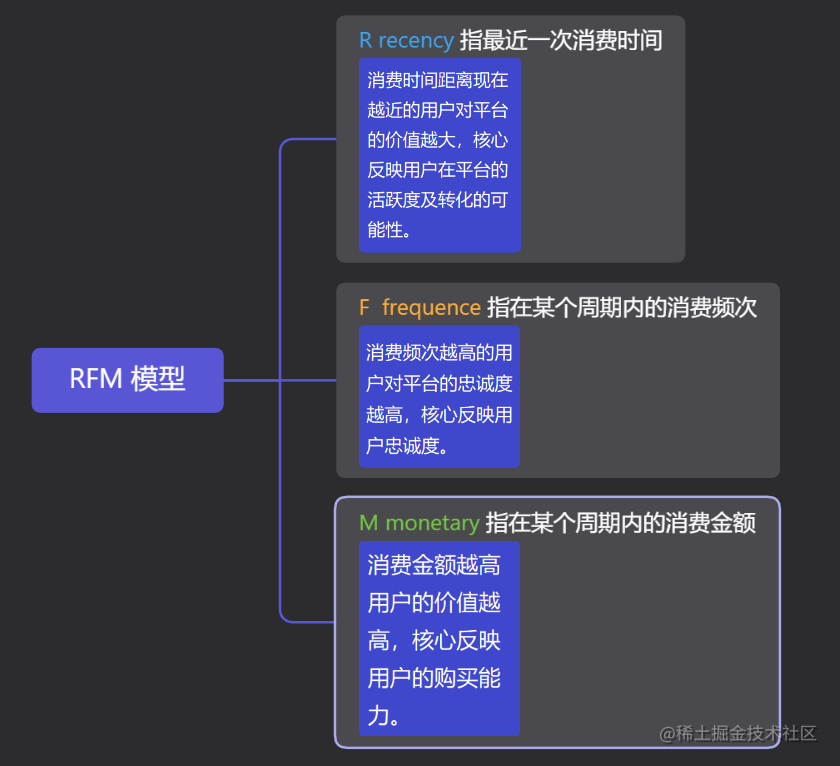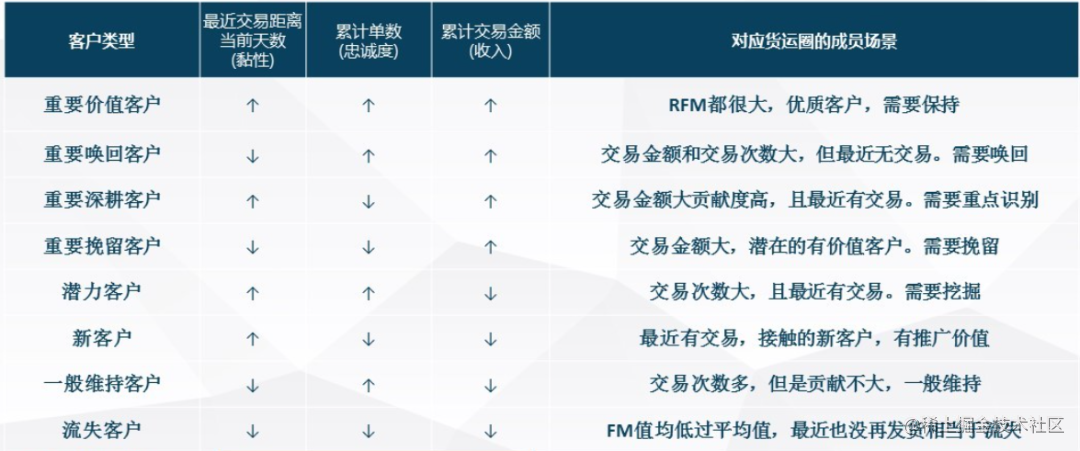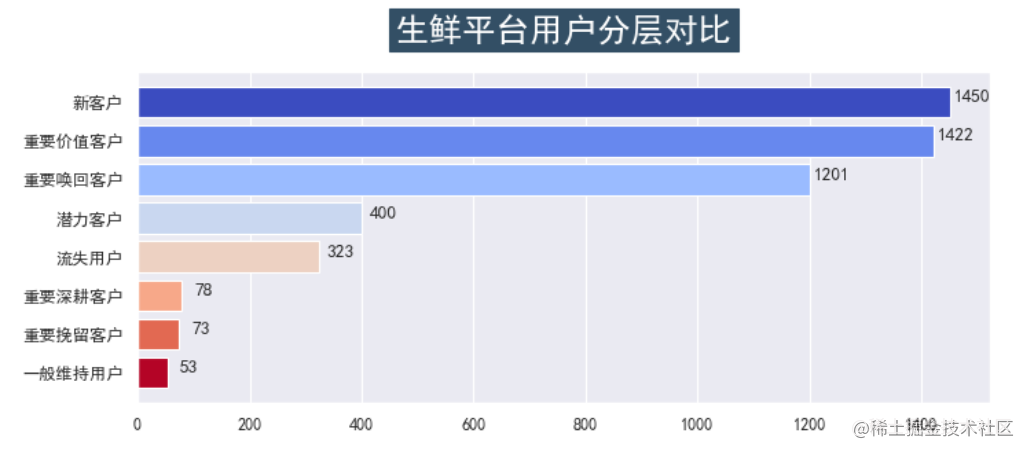### 三、利用 Python 实现 RFM 用户分层

#### 3.1 获取数据

``````import pandas as pd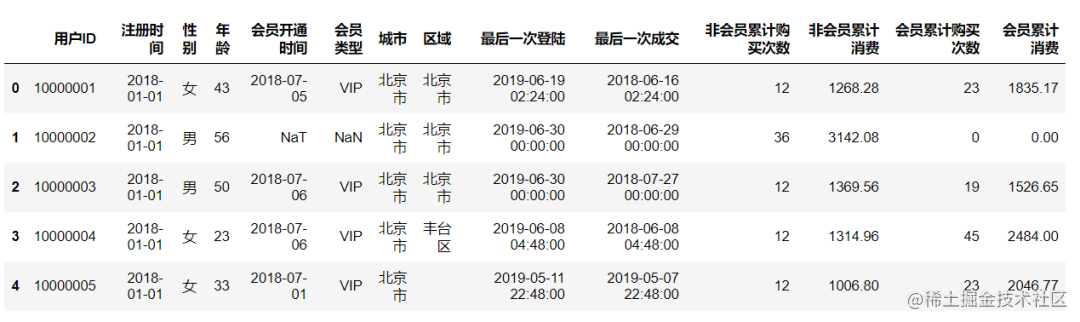``````data.info()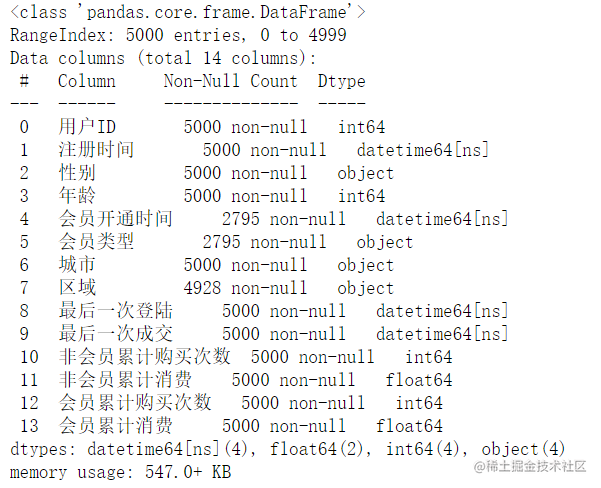``````data.sort_values(by='最后一次成交', ascending=False)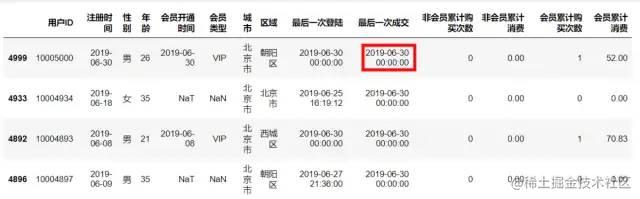#### 3.2 数据处理

``````#  活动结束时间  2019-06-30
data['最后一次成交']=data['最后一次成交'].astype('str')
stop_date = pd.to_datetime('2019-06-30')
datas = data.drop(columns=['注册时间','会员开通时间','会员类型','城市','区域','最后一次登陆'])
datas['最后一次成交时间'] = datas['最后一次成交'].apply(lambda x:x.split())

datas['最后一次成交时间'] = pd.to_datetime(datas['最后一次成交时间'])

datas['R1'] = datas['最后一次成交时间'].apply(lambda x:stop_date-x)

datas['F1'] = datas['非会员累计购买次数']+datas['会员累计购买次数']

datas['M1'] = datas['非会员累计消费'] + datas['会员累计消费']
datas['R1']= datas['R1'].astype(str)
datas['R1']= datas['R1'].apply(lambda x:x.split())
datas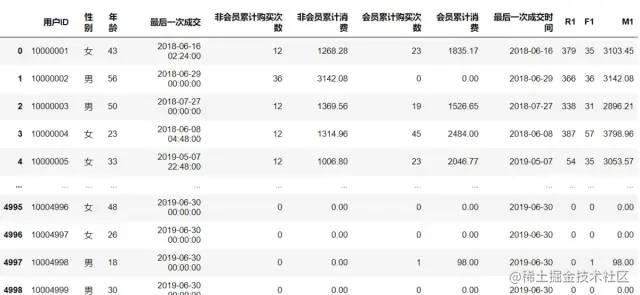#### 3.3 建立模型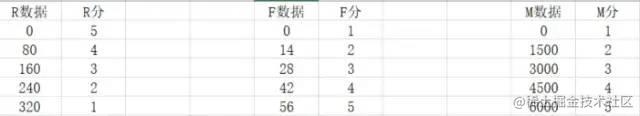``````def R_score(n):
n = int(n)
if 0<n<=80:
r = 5
elif 80<n<=160:
r = 4
elif 160<n<=240:
r = 3
elif 240<n<=320:
r = 2
else:
r = 1
return r

def F_score(n):
n = int(n)
if 0<n<=14:
r = 1
elif 14<n<=28:
r = 2
elif 28<n<=42:
r = 3
elif 42<n<=56:
r = 4
else:
r = 5
return r

def M_score(n):
n = int(n)
if 0<n<=1500:
r = 1
elif 1500<n<=3000:
r = 2
elif 3000<n<=4500:
r = 3
elif 4500<n<=6000:
r = 4
else:
r = 5
return r

datas['M1_score'] =datas['M1'].apply(M_score)
datas['F1_score'] =datas['F1'].apply(F_score)
datas['R1_score'] =datas['R1'].apply(R_score)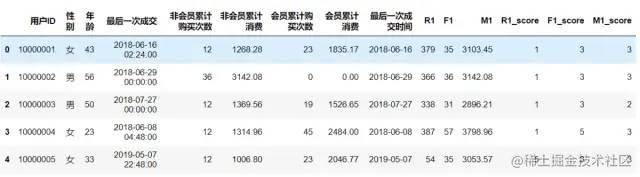``````R_mean = datas['R1_score'].mean()
F_mean = datas['F1_score'].mean()
M_mean = datas['M1_score'].mean()

datas['R'] = datas['R1_score'].apply(lambda x: 1 if x> R_mean else 0)

datas['F'] = datas['F1_score'].apply(lambda x: 1 if x> F_mean else 0)

datas['M'] = datas['M1_score'].apply(lambda x: 1 if x> M_mean else 0)
datas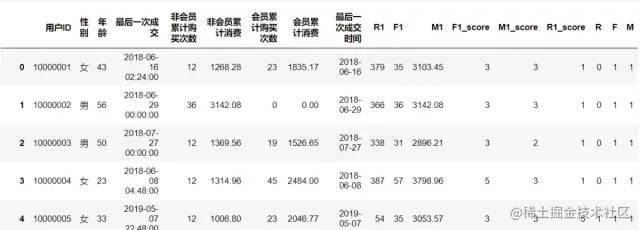``````datas['RFM'] = datas['R'].apply(str)+datas['F'].apply(str)+datas['M'].apply(str)
datas

def user_tag(rfm):
if rfm=='000':
res = '流失用户'
elif rfm=='010':
res = '一般维持用户'
elif rfm=='100':
res = '新客户'
elif rfm=='110':
res = '潜力客户'
elif rfm=='001':
res = '重要挽留客户'
elif rfm=='101':
res = '重要深耕客户'
elif rfm=='011':
res = '重要唤回客户'
else:
res = '重要价值客户'
return res
datas['user_tag']=datas['RFM'].apply(user_tag)
datas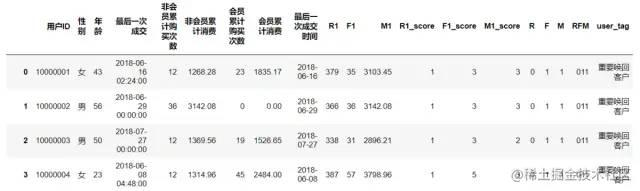#### 3.4 数据可视化

``````import matplotlib.pyplot as plt
import seaborn as sns
import matplotlib as mpl
sns.set(font='SimHei',style='darkgrid')

user_tag = datas.groupby(datas['user_tag']).size()

plt.figure(figsize = (10,4),dpi=80)

user_tag.sort_values(ascending=True,inplace=True)

plt.title(label='生鲜平台用户分层对比',
fontsize=22, color='white',

s = plt.barh(user_tag.index,user_tag.values , height=0.8, color=plt.cm.coolwarm_r(np.linspace(0,1,len(user_tag))))
for rect in s:
width = rect.get_width()
plt.text(width+40,rect.get_y() + rect.get_height()/2, str(width),ha= 'center')

plt.grid(axis='y')
plt.show()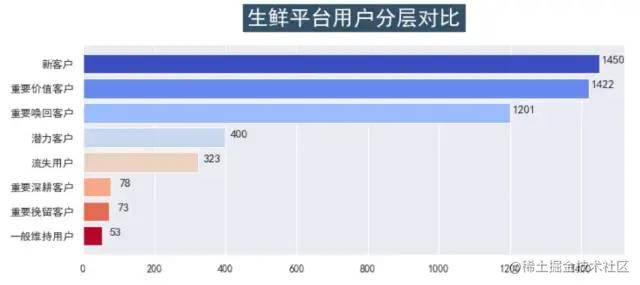``````groups_b = datas.groupby(by='user_tag').size()

plt.figure(figsize = (10,6),dpi=80)
plt.title(label='生鲜平台用户分层占比',
fontsize=22, color='white',

explodes = [0.6, 0, 0, 0, 0,0,0.4,0.8]

patches, l_text, p_text = plt.pie(groups_b.values,labels = groups_b.index, shadow=True,colors=plt.cm.coolwarm_r(np.linspace(0,1,len(groups_b))), autopct='%.2f%%', explode=explodes,startangle=370)
plt.legend(ins,bbox_to_anchor=(2, 1.0))
plt.show()